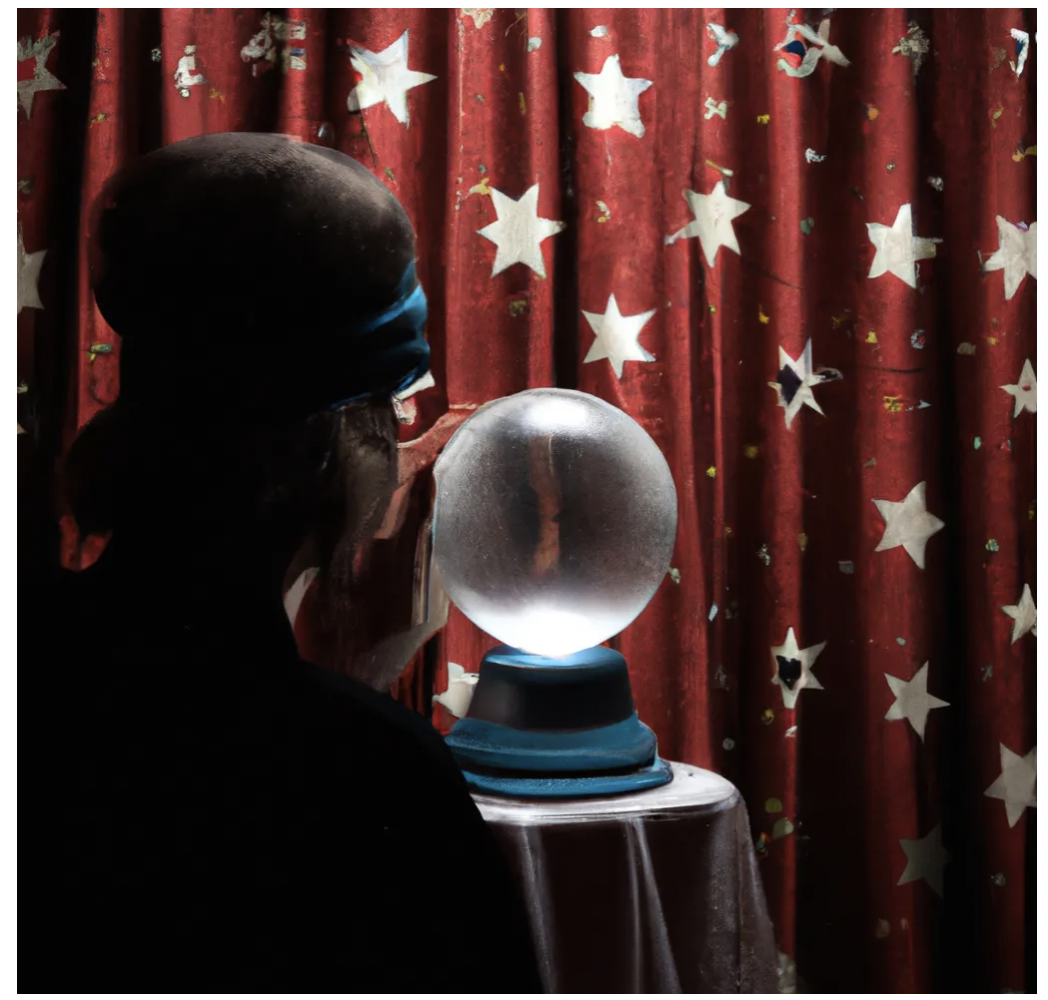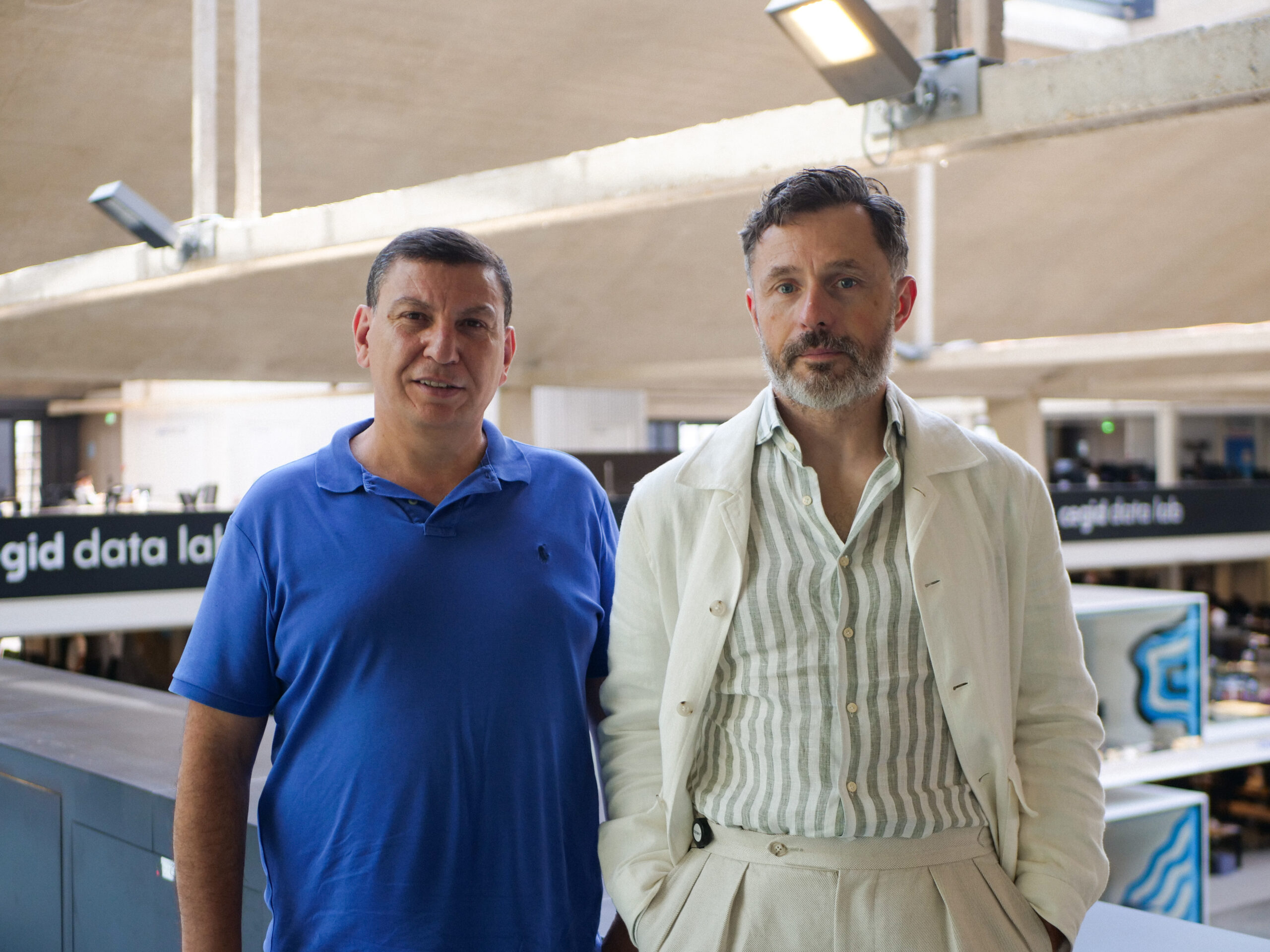# Contextuality in quantum computing

By
Henri de Boutray
January 9, 2023
8 minutesQuantum Mechanics is known as a counter intuitive field, where things seen as impossible in our day-to-day life are perfectly normal. The two examples commonly given when mentioning this counter intuitive aspect of quantum mechanics are the superposition (the fact that Schrödinger’s cat is both dead and alive as long as its box isn’t opened [Sch35]) and entanglement (the fact that two particles can be in a state where acting on one of them would seemingly immediately have an effect of the other, such as measuring one of the qubits of the Bell state [EPR35]). Other properties, such as the destructive nature of the quantum measure (also related to Schrödinger’s cat thought experiment) can be mentioned, but today, I will present you a property that is less popular, but as disconcerting the first time you cross its path: the contextuality!

Contextuality is linked to another notion called locality (itself linked to entanglement) as explored in [AB11] and to hidden variable theories, but we will focus here on a fresh look on the subject by trying to start from an easy to grasp common ground, and introducing only strictly necessary notion as we go.

So! We call a situation contextual when its protagonists need a knowledge of the context in order to explain the aftermath of the situation. And we also need to restrict the ability of the protagonists to only perform local actions (no faster than light communication). Given this, let us examine a case where we would like to see if the situation is contextual or not.

Let us say that we are very worried about a loved one who is at the other end of the world, potentially doing dangerous activities. In order to get reassured, we go and see a fortune teller which would help us see our loved one and make sure he/she is doing OK. The fortune teller welcomes us with a cryptic sentence “Your friend is eating your lunch”. At this point, you remember that you forgot the lunch you just bought on the counter of the kitchen, with your dog (Milly). It seems like the fortune teller has a non localknowledge of the world: he knows something that happened at a distance and this without communication with the remote event. This said, you realize that it’s 11:30, you still have your lunch ticket peeking out of your pocket, and you have Milly’s hairs on your trousers. These three elements are part of the context of the current situation, and this is probably how the fortune teller guessed what happened (or rather what is happening). So in the end the experience you just lived could be explained by a contextual explanation of the event, and the fortune teller has likely no non local abilities.

## 1. Formal definition

After this wacky example of contextuality in our classical world, let’s come back on a quantum definition of it. First, some useful notions: The Pauli matrices are

and we will also use the 2 by 2 identity matrix I and the n by n matrix Id.

We consider a thought experiment where we perform operations on a nqubits system, and I will show you that this thought experiment exhibits a contextual behavior. The experiment is composed of several series of measurements using the operators of the Pauli group. These operators are defined as a Pauli measurement on each wire, with eventually a global phase. They are denoted by

with s ∈ {±1, ±i} and Pᵢ ∈ {X, Y, Z, I}. The result of such measurements is in ±1, and the overall result of the experiment is the product of all measurement result. I will show you in Sec. 3 that we can construct such experiments where quantum theory can produce a result non reproducible by classical, non contextual theory.

## 2. Link with other quantum specific properties

What we call quantum properties are quantum specific behaviors. They can arise directly from the laws of quantum mechanics, such as the destructive nature of measurements, but they can also be “meta” properties , arising from the combination of several other quantum properties. Entanglement is the example of such a meta property (we couldn’t have entanglement without superposition), and contextuality is too: the way quantum measurements work is absolutely necessary for quantum contextuality (the fact that the measure projects the state on the space corresponding to the result observed is the root of the contextual behaviors).

Understanding the links between these properties may help us build an intuition concerning quantum computation. This is likely one of the reasons why they are so studied! In that regard, let us have a look at one of the papers revealing one of the deepest links in my opinion: the 2011 paper by S. Abramsky and A. Brandenburger [AB11].

In this paper, the authors show that non locality and quantum contextuality are in fact two ways to look at the same situation. And in a much deeper level, that some of the concepts having a role in those meta properties are in fact not necessarily related to quantum mechanics specifically but, in a more general way, to the mathematical specificities of the probability distribution of the associated measurement results.

## 3. Link with finite geometries

As teased in Sec. 1, this contextuality property can be exhibited using measures on a n qubit system. The corresponding thought experiment is decomposed as such: on this n qubit system, we will perform several series of measurements. If we represent each operator used for a measurement by a dot, and each series of measurement by a line, we obtain what we call a finite geometry.

This formalism can be complexified such that the finite geometry would live in a vector space, and this allows us to perform interesting operations on those geometries, but I will leave that as a teaser for an upcoming Medium article (for more information, you may read [dBHG+22]).

Fig. 1 represent such an experiment, where the (2 qubits) operators used are the points and the series of measurement are the lines (vertical and horizontal) of this figure. Note that the last vertical line is doubled, we will see shortly why that is.

First, let us check what the result of this experiment will be. Each operator here has its eigenvalues in ±1, meaning each result of a single measurement will either be 1 or −1. In addition, each line is built such that the operators composing it commute (for two operators on a line O₁ and O₂, OO₂ = OO₁). With the rules of quantum mechanics, this results in the fact that the product of the measurements is in the spectrum (the set of all eigenvalues) of the product of the operators (if rᵢ is the result of a measure by
Oᵢ, ∏ᵢ rᵢ ∈ sp(∏ᵢ Oᵢ)).
Given that each line was also made such that the product of the operators is either Id or −Id (and that sp(Id) ={1}), the product of the measures on each line will either be 1 or −1. This is where our doubled line mentioned earlier comes into play: it is the only one where the product of the result is −1. This means that the overall product is −1 and this result cannot be reproduced by classical non contextual local theories!

The reason why this result cannot be reproduced is that such a theory can be modeled by a function that would attribute to each point a value in ±1 (the result of the “measurement”). Each point being in two lines, the product of all measurements would in this case look like
∏ᵢ f(Oᵢ)×∏ᵢ f(Oᵢ) = (∏ᵢ f(Oᵢ))² = 1.
So in one case we obtain as a result -1 while in the other, we can’t ever obtain anything but 1.

As you may have picked up, not all experiments similar to the one shown in Fig. 1 can exhibit this contextual behavior, the operators and series of measurements have to be carefully chosen.

## 4. Link with quantum programs verification

Program verification is a concept that stems as early as from the beginning of computer science. For instance, Hoare logic [Hoa69] was introduced in 1969 whereas the now very famous C language was introduced in 1972! The idea behind program verification is to prove that the program written by the programmer does what it was conceived to do. This proof is possible because a program is in fact nothing but a series of logical instruction, so it can be translated as a series of application of a (mathematical) function to a certain variable encoding the state of the system. The proof is then to show that the output variable validates the condition imposed by the programmer, given the input variable. Let us take a small example to explore this concept:

The program is supposed to compute 2n+1, the condition for the input variable (also called pre-condition) being that it’s a number, the condition for the output (also called post-condition) will be that it’s a number and that program(n) = 2n+1. Given that one can show that we should have 2n+1 = f₂(a) = 2a, and thus that 2n+1 = 2f₁(n) = 2(n+1). Since this is false, we showed that our function has a bug (without executing it)!

This is a very powerful way to verify programs, but it is pretty hard to accomplish. My previous works put the focus on trying to ease this for quantum program, by studying properties of quantum states that could be used in quantum program verification. Contextuality is one of those properties, even if (as of now) it wasn’t used in this context.

## At ColibrITD

At ColibrITD, we create programs that efficiently solve our costumers’ industrial problems. Since we target industrial problems, bugs can be very costly, so having tools to automate the verification of our quantum programs is precious for us!

## References

[AB11] Abramsky, S., and A. Brandenburger. 2011. “The Sheaf-Theoretic Structure of Non-Locality and Contextuality.” New Journal of Physics 13 (11): 113036. https://doi.org/10.1088/1367-2630/13/11/113036.

[dBHG+22] Boutray, H. de, F. Holweck, A. Giorgetti, P. Masson, and M. Saniga. 2022. “Contextuality Degree of Quadrics in Multi-Qubit Symplectic Polar Spaces.” Journal of Physics A: Mathematical and Theoretical 55 (47): 475301. https://doi.org/10.1088/1751-8121/aca36f.

[EPR35] Einstein, A., B. Podolsky, and N. Rosen. 1935. “Can Quantum-Mechanical Description of Physical Reality Be Considered Complete?” Physical Review 47 (10): 777–80. https://doi.org/10.1103/PhysRev.47.777.

[Hoa69] Hoare, C. A. R. 1969. “An Axiomatic Basis for Computer Programming.” Communications of the ACM 12 (10): 576–80. https://doi.org/10.1145/363235.363259.

[Sch35] Schrödinger, E. 1935. “Die gegenwärtige Situation in der Quantenmechanik.” Naturwissenschaften 23 (48): 807–12. https://doi.org/10.1007/BF01491891.

 this is not a standard name in this contextHenri de Boutray